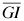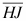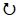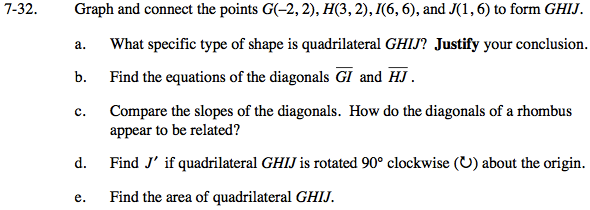### Home > GC > Chapter 7 > Lesson 7.1.3 > Problem7-32

7-32.
1. Graph and connect the points G(−2, 2), H(3, 2), I(6, 6), and J(1, 6) to form GHIJ. 7-32 HW eTool (Desmos).Homework Help ✎

1. What specific type of shape is quadrilateral GHIJ? Justify your conclusion.

2. Find the equations of the diagonalsand.

3. Compare the slopes of the diagonals. How do the diagonals of a rhombus appear to be related?

4. Find J′ if quadrilateral GHIJ is rotated 90° clockwise () about the origin.

5. Find the area of quadrilateral GHIJ.Use the Pythagorean Theorem and the triangles shown to determine the lengths of GJ and HI.